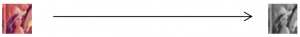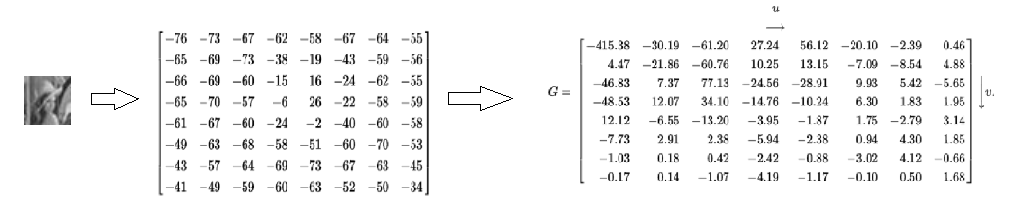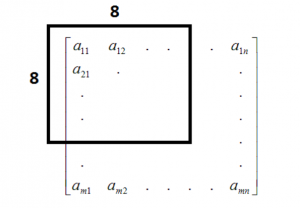Perceptual hashing? Has anyone ever heard about it? Not many people, I think, although it’s a really interesting and useful algorithm. Before I get to the point I strongly recommend reading the following terms which can help you with understanding the Perceptual Hashing algorithm implementation:

1. Hash function
2. Average hashing
3. Hamming distance
4. Discrete cosine transform

Perceptual Hashing is an algorithm which converts various forms of multimedia (i.e. video, audio, image) to a hash format. The most important difference between a common hash function is the fact that during the execution of the Perceptual Hash function for two very similar input hashes will be very close to each other in contrast to the usual hash function. This property is to be applied to finding cases online of copyright infringement. Additionally, the algorithm is resistance to simple modifications like shifts, rotations or noises which can be considered a way to calculate image similarity in some cases.

Perceptual Hashing algorithm steps for images:

1. Image scaling
Reduce original image to small size e.g 32×32 px using one of scaling algorithm.2. Greyscale transformation
Transform the reduced image to a grey scale using one of the greyscale conversion method.3. Discrete cosine transform
Key step. The previous step causes each pixel to be represented as one value, hence, the image can be represented as a two-dimensional matrix. So now we can compute the DCT of the image following Wikipedia description.4. Left-top block
Take the 8×8 (this parameter can be changed) left-top block of output from the previous step.5. Calculate average
From the block taken earlier, calculate the average of all values skipping the first one.6. Calculate the bits
Take the output matrix from step 4 and the average from step 5. Next, set the bits in the following way: if the value is greater then the calculated average in the previous step then set 1 if less than 0.
7. Create hash
Calculated bit sequence can convert to Big Endian system.

To sum up, Perceptual Hashing is a very interesting algorithm which can be used in many various cases, not only for copyright infringement. I recommend tweaking it and keeping in mind when you run into an image similarity problem.